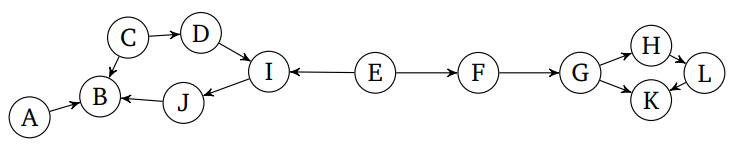# GATE | Sudo GATE 2020 Mock III (24 January 2019) | Question 25

Which of the following is a topological sort of the following graph:(A) E, F, H, G, L, K, A, C, D, I, J, B
(B) E, F, G, H, L, K, A, D, C, I, J, B
(C) E, F, G, H, L, K, A, C, D, I, J, B
(D) E, F, G, H, L, K, B, C, D, I, J, A